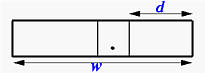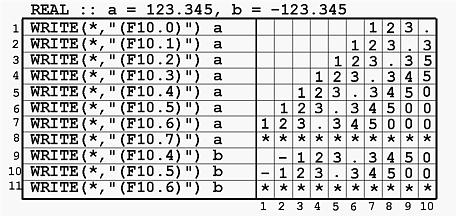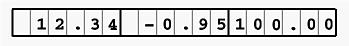# REAL Output: The F DescriptorThe Fw.d descriptor is for REAL output. The general form is:

rFw.d

The meaning of r, w and d are:

• F is for REAL
• w is the width of field, which indicates that a real number should be printed with w positions.
• d indicates the number of digits after the decimal point. More precisely, of these w positions, the right-most d positions are for the fraction part of a real number, and the d+1 position from the right is a decimal point. The remaining w-(d+1) positions are for the integral part. This is shown in the figure below:• When a real number is printed, its integral part is printed as if it uses Im-(d+1). Therefore, if the number of positions is not enough to print the integral part completely, all w positions will be filled with asterisks. Please note that the integral part contains a sign and as a result w should be greater than or equal to d+2.
• The fractional part may have more than d digits. In this case, the (d+1)th digit will be rounded to the dth one. For example, if 1.73 is printed with F3.1 (using 3 positions to print a real number with the right-most position for the fractional part), since the second digit of the fractional part is 3, the result is 1.7. However, if 1.76 is printed with F3.1, the result becomes 1.8 because 6 is rounded.
• The fractional part may have fewer than d digits. In this case, trailing zeros will be added.
• Note that d can be zero. In this case, no fractional part will be printed. More precisely, the right-most position is the decimal point.
• r is the repetition indicator, which gives the number of times the edit descriptor should be repeated. For example, 3F5.3 is equivalent to F5.3, F5.3, F5.3.### Examples

Let us look at the following example. There are two REAL variables a and b with values 123.345 and -123.345, respectively. In the following table, the WRITE statements are shown in the left and their corresponding output, all using five positions, are shown in the right.• The first line prints 123.345 with F10.0. This means that of the ten positions the right-most one should contain a decimal point (since d is zero). Therefore, no fractional part is printed.
• The second line is easy.
• The third line uses F10.2 to print 123.345. Of these ten positions, the last two are used to print the fractional part (i.e., 345). Therefore, the third digit, 5, is rounded yielding 35. Hence the result is 123.35.
• The fourth line uses F10.4 to print 123.345. Since there are four positions for the fractional part, which is larger than the number of actual digits, a trailing zero is added.
• The eighth line uses F10.7 to print 123.345. Since there are only 10-(7+1)=2 positions for printing the integral part which has three digits, all 10 positions are filled with asterisks.
• The eleventh line has the same result. There are 10-(6+1)=3 positions for the integral part. However, the integral part -123 requires four positions. As a result, all 10 positions are filled with asterisks.
Consider the following example. The WRITE statement has three REAL variables and consequently the format must also have three F edit descriptors, one for each variable.

```REAL :: a = 12.34, b = -0.945, c = 100.0

WRITE(*,"(3F6.2)")  a, b, c
```
The edit descriptor is 3F6.2 and the format is equivalent to (F6.2,F6.2,F6.2) because the repetition indicator is 3. Therefore, each of these three REAL variables is printed with F6.2. Based on the discussion earlier, the result is the following: